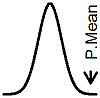P.Mean: What's the name of the test for comparing two proportions? (created 2012-09-12). News: Sign up for "The Monthly Mean," the newsletter that dares to call itself average, www.pmean.com/news.A commonly used statistical test is the comparison of two independent proportions. For example, you are looking at the rate of steroid induced hyperglycemia among patients receiving high doses of steroids compared to the rate among patients receiving low doses. There are several terms that you can use here because there are several equivalent ways to test this hypothesis. I prefer to refer to the statistical method here as logistic regression. Here's why.

The comparison of two proportions can be done using a normal approximation. The test statistic has the formthough the formula that you see might use slightly different notation. You compare this test statistic to a percentile from a standard normal distribution. There are several names for this test. It could be called the "z test of two independent proportions" although wording with terms like "normal approximation" added or substituted would also be okay.

You can also arrange the data into a two by two table and calculate a chi-squared statistic. The formula for this test isand again, the formula you have seen might be a slight variation. You compare this test statistic to a percentile from a chi-squared distribution with one degree of freedom. This is the "chi-squared test of two independent proportions." You can just call it a "chi-squared test" though that have a fair amount of ambiguity. There are many other tests out there that go by a closely related name, such as the chi-squared test of goodness of fit.

By the way, there are many variations on the word "chi-squared." Some people run the two words together (chisquared) and some make it two separate words (chi squared). Some drop the "d" on the end (chi-square). Some will capitalize the first letter (Chi-squared). If you count up the variations, there are at least a dozen versions in common use. I myself am not consistent, and I really don't think it matters all that much.

It turns out that the two tests are identical when you have a two sided hypothesis. For a one-sided hypothesis, you have to use the z test.

You can also put this data into a logistic regression model. I'm not sure if this exactly identical to the previous two tests, but if not, it is very close. You might think that logistic regression is overkill for this data, but I like it because it leaves open the option for adjusting for other covariates in the model. Also, it allows you to consider continuous alternatives that are not available in the z or chi-squared tests. For example, instead of comparing high doses to low doses, you could fit a model with some function of the actual dose. It might be linear on a log-odds scale or it might not, but it is certainly worth investigating.

When you say "logistic regression," you are leaving the door open for a wider variety of additional analysis. That's why I prefer this term over "z test" or "chi-squared test."This page was written by Steve Simon and is licensed under the Creative Commons Attribution 3.0 United States License. Need more information? I have a page with general help resources. You can also browse for pages similar to this one at Writing Research Papers.